# RD Sharma Class 8 Solutions Chapter 3 Squares and Square Roots Ex 3.3

In this chapter, we provide RD Sharma Class 8 Solutions Chapter 3 Squares and Square Roots Ex 3.3 for English medium students, Which will very helpful for every student in their exams. Students can download the latest RD Sharma Class 8 Solutions Chapter 3 Squares and Square Roots Ex 3.3 Maths pdf, free RD Sharma Class 8 Solutions Chapter 3 Squares and Square Roots Ex 3.3 Maths book pdf download. Now you will get step by step solution to each question.

### RD Sharma Solutions for Class 8 Chapter 3 Squares and Square Roots Ex 3.3 Download PDF

Question 1.
Find the squares of the following numbers using column method. Verify the result by finding the square using the usual multiplication :
(i) 25
(ii) 37
(iii) 54
(iv) 71
(v) 96
Solution:
(i) (25)2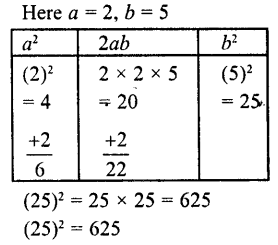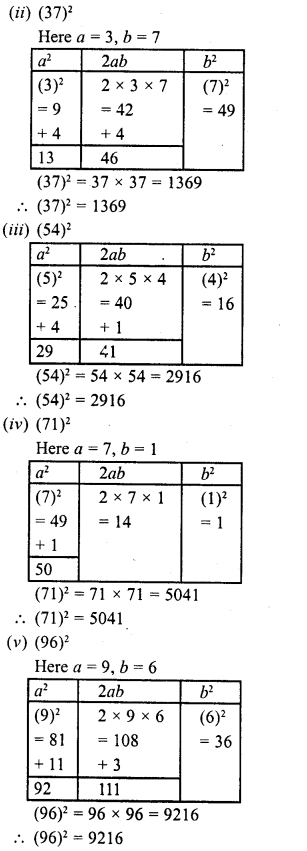Question 2.
Find the squares of the following numbers using diagonal method :
(i) 98
(ii) 273
(iii) 348
(iv) 295
(v) 171
Solution: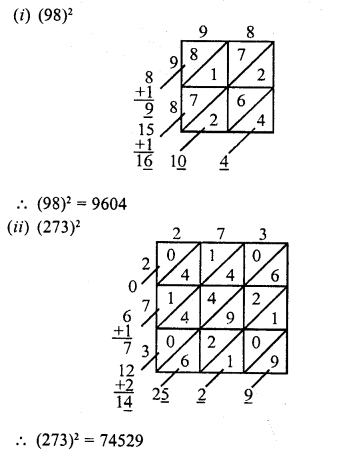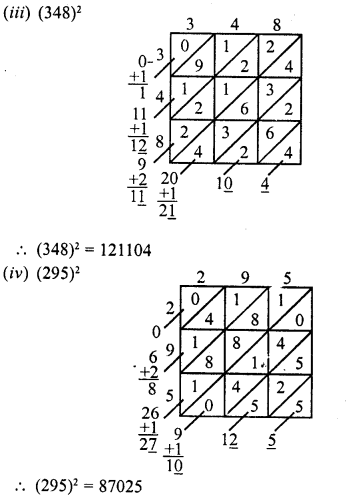Question 3.
Find the squares of the following numbers :
(i) 127
(ii) 503
(iii) 451
(iv) 862
(v) 265
Solution:
(i) (127)2 = (120 + 7)2
{(a + b)2 = a2 + lab + b2}
= (120)2 + 2 x 120 x 7 + (7)2
= 14400+ 1680 + 49 = 16129

(ii) (503)2 = (500 + 3)2
{(a + b)2 = a2 + lab + b1}
= (500)2 + 2 x 500 x 3 + (3)2
= 250000 + 3000 + 9 = 353009

(iii) (451)2 = (400 + 51)2
{(a + b)2 = a2 + lab + b2}
= (400)2 + 2 x 400 x 51 + (5l)2
= 160000 + 40800 + 2601 = 203401

(iv) (451)2 = (800 + 62)2
{(a + b)2 = a2 + lab + b2}
= (800)2 + 2 x 800 x 62 + (62)2
= 640000 + 99200 + 3844 = 743044

(v) (265)2
{(a + b)2 = a2 + 2ab + b2}
(200 + 65)2 = (200)2 + 2 x 200 x 65 + (65)2
= 40000 + 26000 + 4225 = 70225

Question 4.
Find the squares of the following numbers
(i) 425
(ii) 575
(iii) 405
(iv) 205
(v) 95
(vi) 745
(vii) 512
(viii) 995
Solution:
(i) (425)2
Here n = 42
∴ n (n + 1) = 42 (42 + 1) = 42 x 43 = 1806
∴ (425)2 = 180625

(ii) (575)2
Here n = 57
∴ n (n + 1) = 57 (57 + 1) = 57 x 58 = 3306
∴ (575)2 = 330625

(iii) (405)2
Here n = 40
∴ n (n + 1) = 40 (40 + 1) -40 x 41 = 1640
∴ (405)2 = 164025

(iv) (205)2
Here n = 20
∴ n (n + 1) = 20 (20 + 1) = 20 x 21 = 420
∴ (205)2 = 42025

(v) (95)2
Here n = 9
∴ n (n + 1) = 9 (9 + 1) = 9 x 10 = 90
∴ (95)2 = 9025

(vi) (745)2
Here n = 74
∴ n (n + 1) = 74 (74 + 1) = 74 x 75 = 5550
∴ (745)2 = 555025

(vii) (512)2
Here a = 1, b = 2
∴ (5ab)2 = (250 + ab) x 1000 + (ab)2
∴ (512)2 = (250 + 12) x 1000 + (12)2
= 262 x 1000 + 144
= 262000 + 144 = 262144

(viii) (995)2
Here n = 99
∴ n (n + 1) = 99 (99 + 1) = 99 x 100 = 9900
∴ (995)2 = 990025

Question 5.
Find the squares of the following numbers using the identity (a + b)1 = a2 + lab + b1
(i) 405
(ii) 510
(iii) 1001
(iv) 209
(v) 605
Solution:
a + b)2 = a2 + lab + b2

(i) (405)2 = (400 + 5)2
= (400)2 + 2 x 400 x 5 + (5)2
= 160000 + 4000 + 25 = 164025

(ii) (510)2 = (500 + 10)2
= (500)2 + 2 x 500 x 10 x (10)2
= 250000 + 10000 + 100
= 260100

(iii) (1001)2 = (1000+1)2
= (1000)2 + 2 X 1000 x 1 + (1)= 1000000 + 2000 + 1
=1002001

(iv) (209)2 = (200 + 9)2
= (200)2 + 2 x 200 x 9 x (9)2
= 40000 + 3600 +81
= 43681

(v) (605)2 = (600 + 5)2
= (600)2 + 2 x 600 x 5 +(5)2= 360000 + 6000       25
=366025

Question 6.
Find the squares of the following numbers using the identity (a – b)2 = a2 – 2ab + b2 :
(i) 395
(ii) 995
(iii) 495
(iv) 498
(v) 99
(vi) 999
(vii) 599
Solution:
a – b)2 = a2 – lab + b2

(i) (395)2 = (400 – 5)2
= (400)2 – 2 x 400 x 5 + (5)2
= 160000-4000 + 25
= 160025-4000
= 156025

(ii) (995)2 = (1000 – 5)2
= (1000)2 – 2 x 1000 x 5 + (5)2
= 1000000- 10000 + 25
= 1000025- 10000
= 990025

(iii) (495)2 = (500 – 5)2
= (500)2 – 2 x 500 x 5 + (5)2
= 250000 – 5000 + 25
= 250025 – 5000
= 245025

(iv) (498)2 = (500 – 2)2
= (500)2 – 2 x 500 x 2 + (2)2
= 250000 – 2000 + 4
= 250004 – 2000
= 248004

(v) (99)2 = (100 – l)2
= (100)2 – 2 x 100 x 1 + (1)2
= 10000 – 200 + 1
= 10001 – 200
= 9801

(vi) (999)2 = (1000- l)2
= (1000)2 – 2 x 1000 x 1+ (1)2
= 1000000-2000+1
= 10000001-2000=998001

(vii) (599)2 = (600 – 1)2
= (600)-2 x 600 X 1+ (1)2
= 360000 -1200+1
= 360001 – 1200 = 358801

Question 7.
Find the squares of the following numbers by visual method :

(i) 52
(ii) 95
(iii) 505
(iv) 702
(v) 99
Solution:
(a + b)2 = a2 – ab + ab + b2
(i) (52)2 = (50 + 2)2
= 2500 + 100 + 100 + 4
= 2704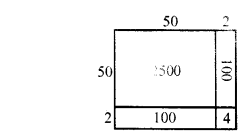(ii) (95)2 = (90 + 5)2
= 8100 + 450 + 450 + 25
= 9025(iii) (505)2 = (500 + 5)2
= 250000 + 2500 + 2500 + 25
= 255025(iv) (702)2 = (700 + 2)2
= 490000 + 1400+ 1400 + 4
= 492804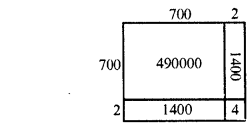(v) (99)2 = (90 + 9)2
= 8100 + 810 + 810 + 81
= 9801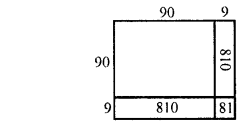All Chapter RD Sharma Solutions For Class 8 Maths

—————————————————————————–

All Subject NCERT Exemplar Problems Solutions For Class 8

All Subject NCERT Solutions For Class 8

*************************************************

I think you got complete solutions for this chapter. If You have any queries regarding this chapter, please comment on the below section our subject teacher will answer you. We tried our best to give complete solutions so you got good marks in your exam.

If these solutions have helped you, you can also share rdsharmasolutions.in to your friends.